# RD Sharma Solutions for Class 9 Maths Chapter 4 Algebraic Identities Exercise 4.1

RD Sharma Class 9 Mathematics Chapter 4 Exercise 4.1 Algebraic Identities is provided here. BYJU’S experts have prepared RD Sharma Class 9 chapter 4 solutions in accordance with CBSE syllabus for class 9. Studying this chapter will help you to gain extra knowledge of algebraic identities. Students can download RD Sharma exercise 4.1 by clicking on the link below.

## Download PDF of RD Sharma Solutions for Class 9 Maths Chapter 4 Algebraic Identities Exercise 4.1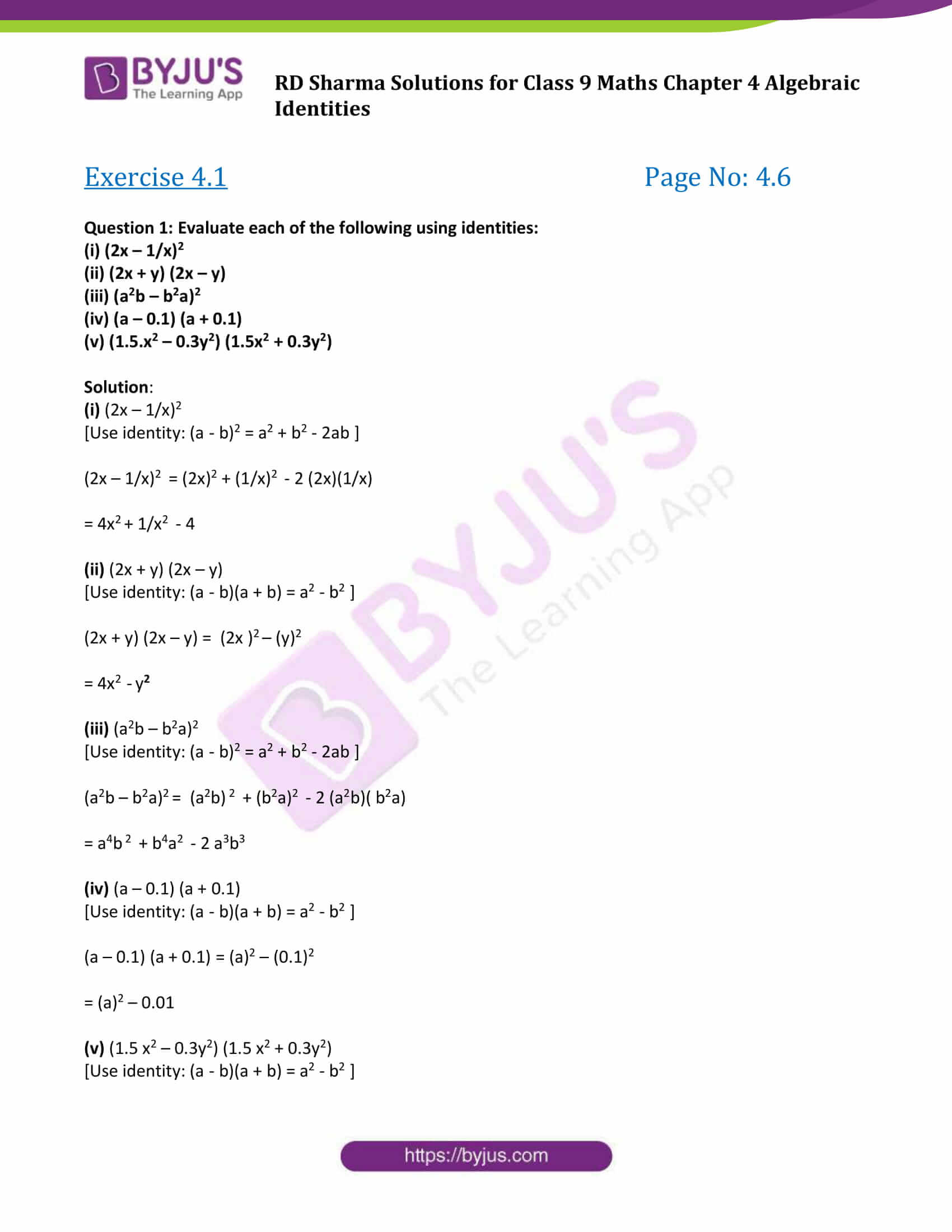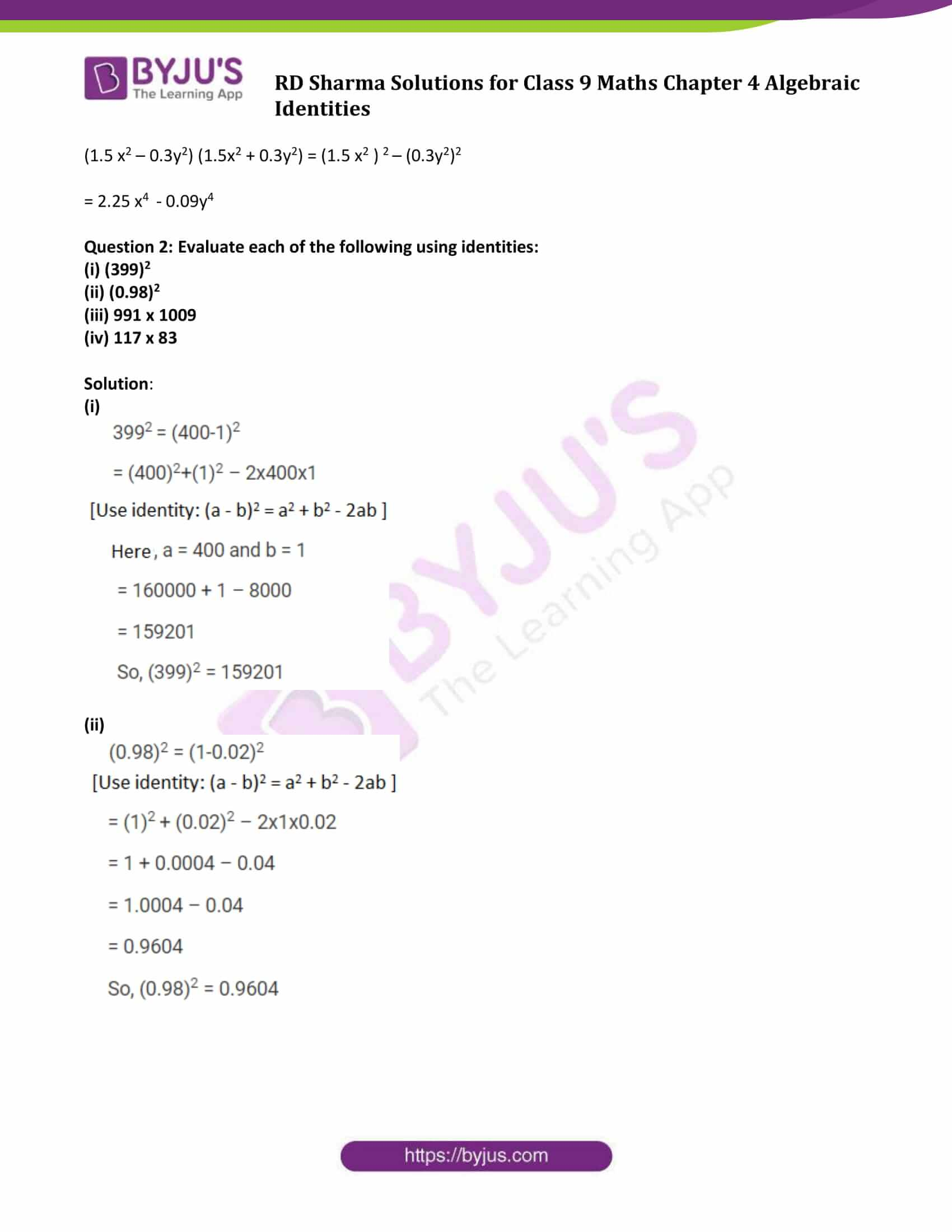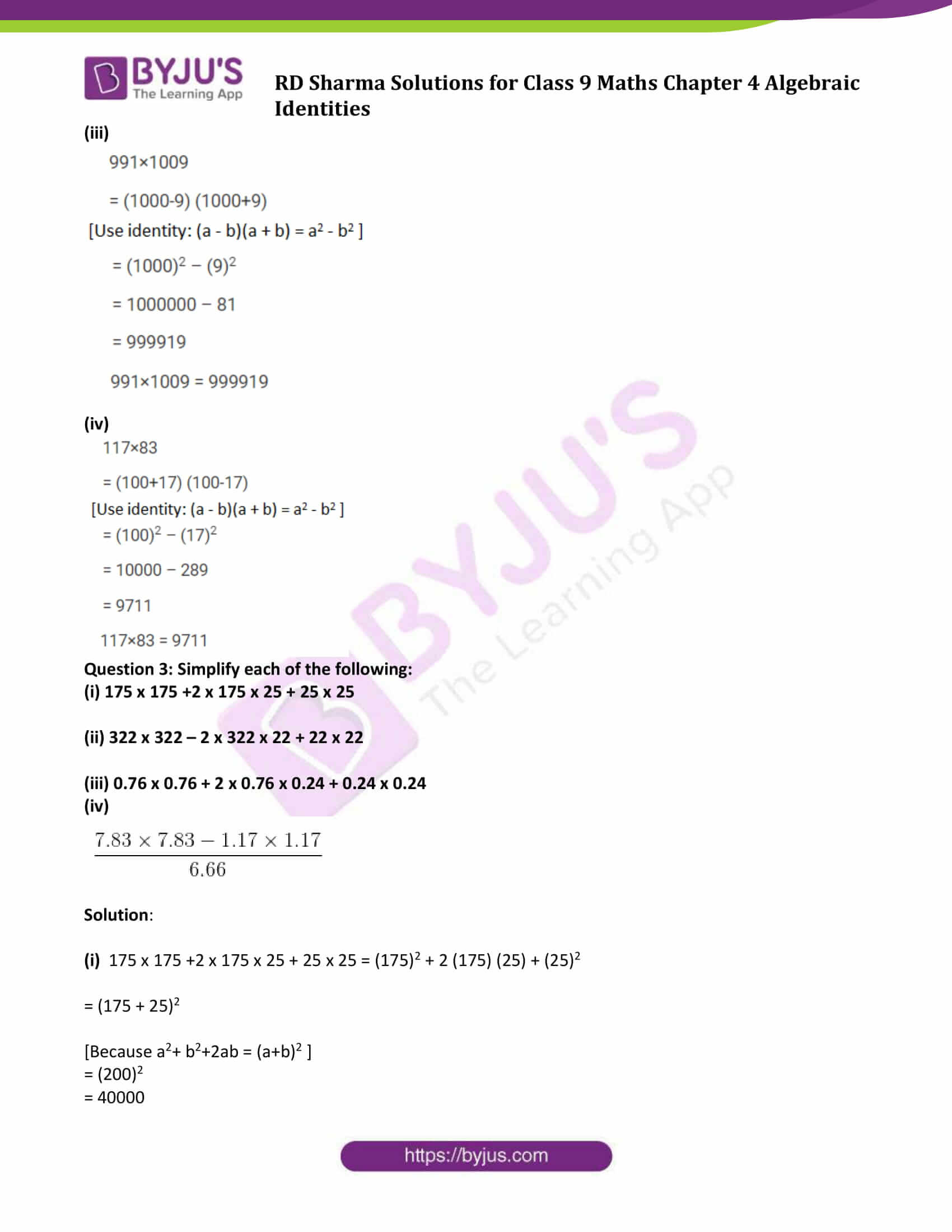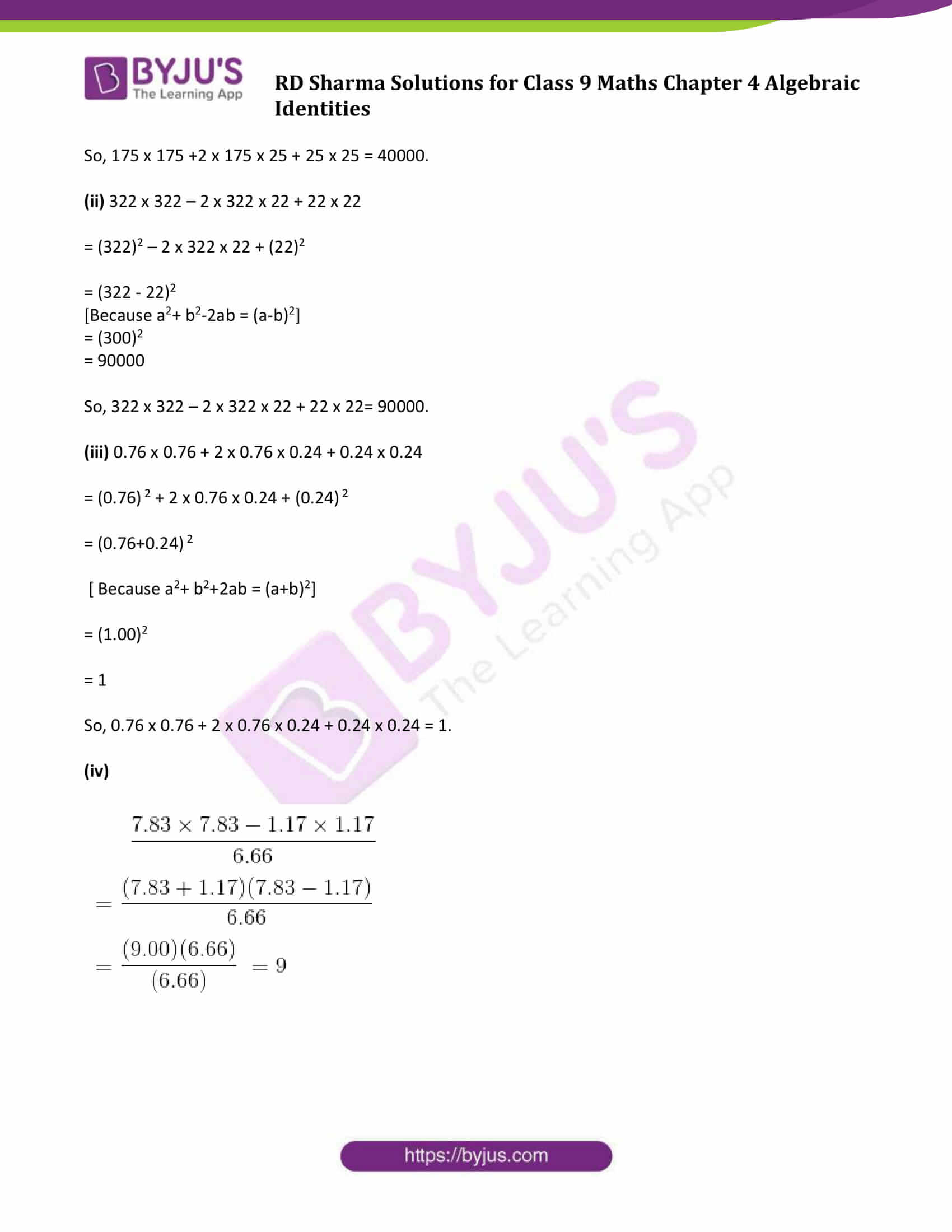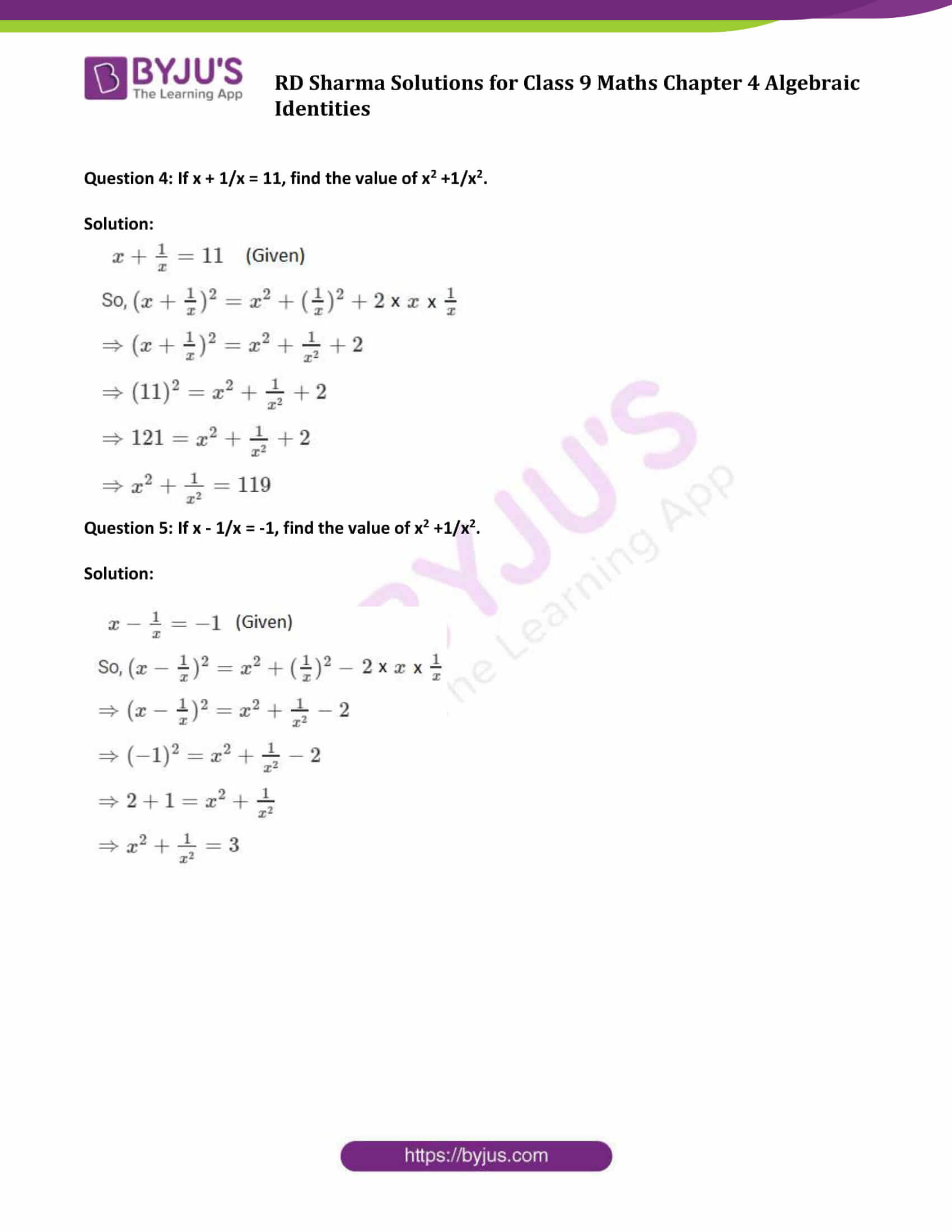### Access Answers to Maths RD Sharma Solutions for Class 9 Chapter 4 Algebraic Identities Exercise 4.1 Page number 4.6

#### Exercise 4.1 Page No: 4.6

Question 1: Evaluate each of the following using identities:

(i) (2x – 1/x)2

(ii) (2x + y) (2x – y)

(iii) (a2b – b2a)2

(iv) (a – 0.1) (a + 0.1)

(v) (1.5.x2 – 0.3y2) (1.5x2 + 0.3y2)

Solution:

(i) (2x – 1/x)2

[Use identity: (a – b)2 = a2 + b2 – 2ab ]

(2x – 1/x)2 = (2x)2 + (1/x)2 – 2 (2x)(1/x)

= 4x2 + 1/x2 – 4

(ii) (2x + y) (2x – y)

[Use identity: (a – b)(a + b) = a2 – b2 ]

(2x + y) (2x – y) = (2x )2 – (y)2

= 4x2 – y2

(iii) (a2b – b2a)2

[Use identity: (a – b)2 = a2 + b2 – 2ab ]

(a2b – b2a)2 = (a2b) 2 + (b2a)2 – 2 (a2b)( b2a)

= a4b 2 + b4a2 – 2 a3b3

(iv) (a – 0.1) (a + 0.1)

[Use identity: (a – b)(a + b) = a2 – b2 ]

(a – 0.1) (a + 0.1) = (a)2 – (0.1)2

= (a)2 – 0.01

(v) (1.5 x2 – 0.3y2) (1.5 x2 + 0.3y2)

[Use identity: (a – b)(a + b) = a2 – b2 ]

(1.5 x2 – 0.3y2) (1.5x2 + 0.3y2) = (1.5 x2 ) 2 – (0.3y2)2

= 2.25 x4 – 0.09y4

Question 2: Evaluate each of the following using identities:

(i)(399)2

(ii)(0.98)2

(iii)991 x 1009

(iv) 117 x 83

Solution:

(i)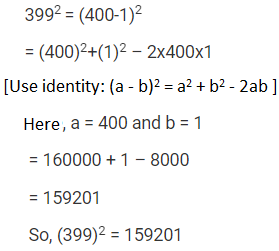(ii)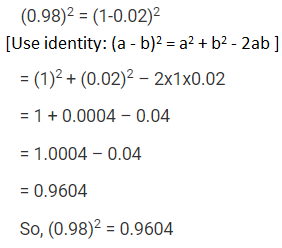(iii)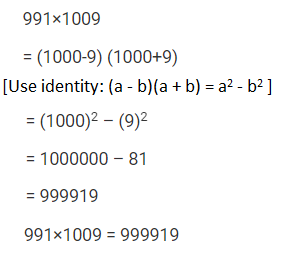(iv)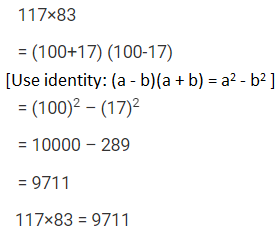Question 3: Simplify each of the following:

(i) 175 x 175 +2 x 175 x 25 + 25 x 25

(ii) 322 x 322 – 2 x 322 x 22 + 22 x 22

(iii) 0.76 x 0.76 + 2 x 0.76 x 0.24 + 0.24 x 0.24

(iv)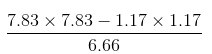Solution:

(i) 175 x 175 +2 x 175 x 25 + 25 x 25 = (175)2 + 2 (175) (25) + (25)2

= (175 + 25)2

[Because a2+ b2+2ab = (a+b)2 ]

= (200)2

= 40000

So, 175 x 175 +2 x 175 x 25 + 25 x 25 = 40000.

(ii) 322 x 322 – 2 x 322 x 22 + 22 x 22

= (322)2 – 2 x 322 x 22 + (22)2

= (322 – 22)2

[Because a2+ b2-2ab = (a-b)2]

= (300)2

= 90000

So, 322 x 322 – 2 x 322 x 22 + 22 x 22= 90000.

(iii) 0.76 x 0.76 + 2 x 0.76 x 0.24 + 0.24 x 0.24

= (0.76) 2 + 2 x 0.76 x 0.24 + (0.24) 2

= (0.76+0.24) 2

[ Because a2+ b2+2ab = (a+b)2]

= (1.00)2

= 1

So, 0.76 x 0.76 + 2 x 0.76 x 0.24 + 0.24 x 0.24 = 1.

(iv)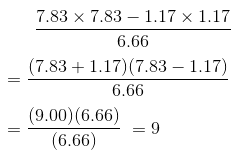Question 4: If x + 1/x = 11, find the value of x2 +1/x2.

Solution: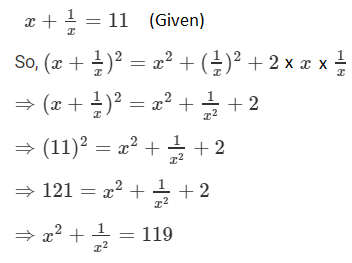Question 5: If x – 1/x = -1, find the value of x2 +1/x2.

Solution: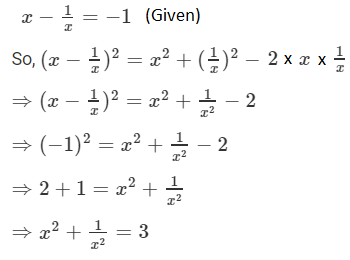## RD Sharma Solutions for Class 9 Maths Chapter 4 Algebraic Identities Exercise 4.1

RD Sharma Solutions Class 9 Maths Chapter 4 Algebraic Identities Exercise 4.1 is based on the following algebraic Identities:

• (a + b)2 = a2 + 2ab + b2
• (a – b)2 = a2 – 2ab + b2
• (a – b)(a + b) = a2 – b2
• (x + a)(x + b) = x2 + (a + b)x + ab